## Sunday, August 21, 2011

### DIY @mention constellations, Part III

So you checked out Part I and Part II, no doubt. You probably installed Graphviz, maybe you tried out the shell script I posted on pastebin, and perhaps you have your own one of these now:If so, nice going. This post is going to dial it wayyy back and start at the beginning working with some basic Graphviz functionality.

Graphviz is a suite of software tools for working with graph data, where you can think of a graph as a set of nodes, some of which are connected by edges. At its most basic a graph is a bag of dots with a bunch of lines connecting some dots to other dots. As you'll see, there's more than one way of representing this visually

But talking of dots, Graphviz works with data assembled into a format known itself as DOT. Here's an example of a graph defined in the DOT language:

``` digraph basic {     x -> y     y -> x     y -> z     z -> a     z -> a     a -> x } ```
It defines a graph where the nodes are identified by the letters x, y, z and a.

Save the above graph definition as a text file called basic.gv (Graphviz documents have extension .gv by convention); we're going to use the basic Graphviz commands to visualize this graph in different ways.

At the command line, run this:

`dot basic.gv -Tpng > basic-dot.png`
and here's what you'll get as output: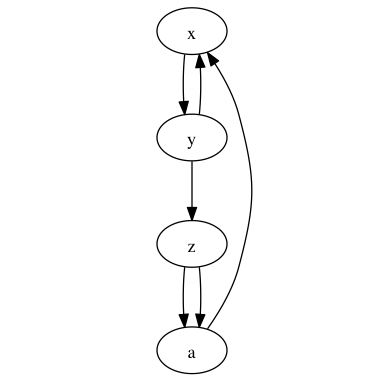Simple! This represents our graph perfectly. Try this:
`neato basic.gv -Tpng > basic-neato.png`
to get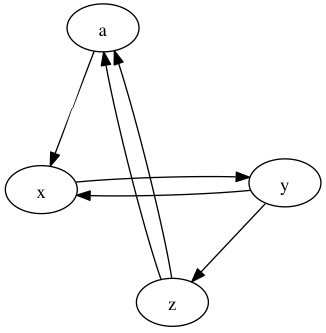And then there's twopi and circo:
`twopi basic.gv -Tpng > basic-twopi.png`
gives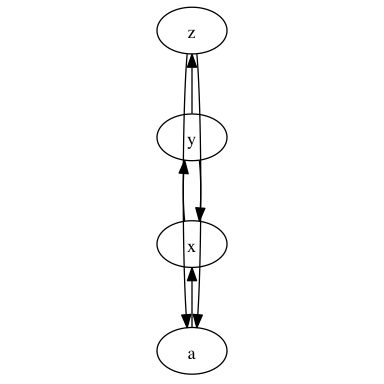and
`circo basic.gv -Tpng > basic-circo.png`
results in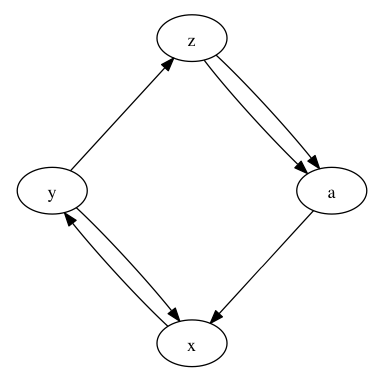Finally, we're going to add some styling options to the graph. Run this command:

`circo basic.gv -Gbgcolor=black -Ecolor=yellow -Earrowsize=0.3 -Epenwidth=0.4 -Nlabel='' -Nwidth=0 -Nheight=0 -Nfixedsize=true -Gsize=4 -Gratio=fill -Tpng > basic-circo-options.png`
to get the output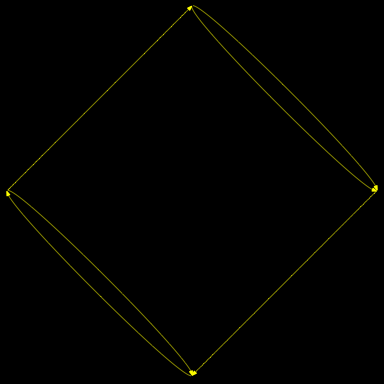You can see how this works. The -G options apply to the whole Graph. The -N options to the Nodes and the -E options to the Edges. There's online documentation covering all the various options.

Now imagine that rather than basic.gv

``` digraph basic {     x -> y     y -> x     y -> z     z -> a     z -> a     a -> x } ```
we have instead a graph representing Twitter users @mentioning each other. In the next post we'll look at how we could apply Graphviz to that.

In the meantime, here's where you can ultimately take this. A 369 megapixel graph of the largest connected component amongst 30m mentions on Twitter, with the most active 80k users removed. I'm working on identifying the clumps; I suspect that they're geographic or language regions.

Next: Part IV